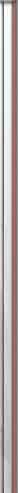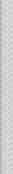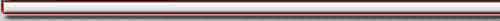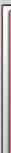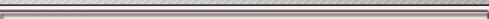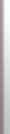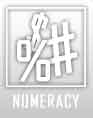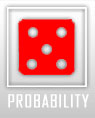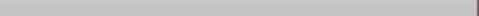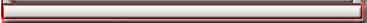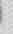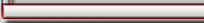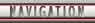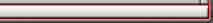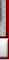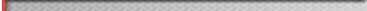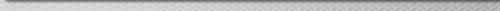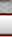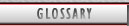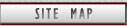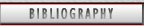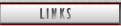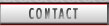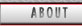PIN » Probability » Probability Home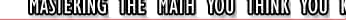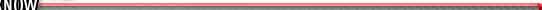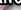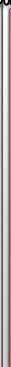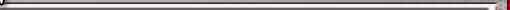Probability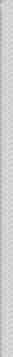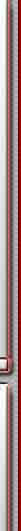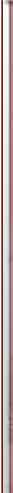Probability is the chance of something happening. It is the measure of how likely it is for an event to occur. When we talk about how probable something is we say things like 'unlikely', 'probably', 'even chance', 'almost definitely' and '100% certain'. The probability of an event is always represented by a number between zero and 1. All these are ways of talking about probability, but how can we use numbers to comprehend probabilities?

Sections:

Next : Probability Scale